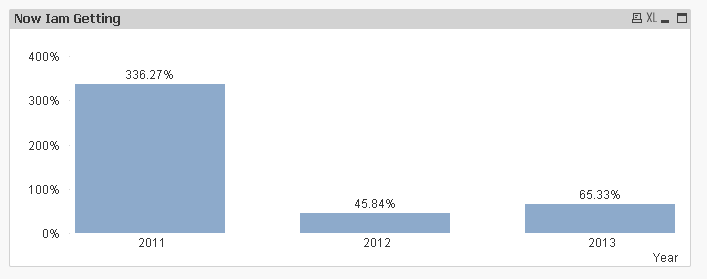# QlikView App Dev

Discussion Board for collaboration related to QlikView App Development.

cancel
Showing results for
Did you mean:Creator III

## How to calculate YTD

Hi Guys,

I have a requirement that i need to calculate YTD for a data

Year To Date (YTD):

Dimensions: Year

I need to show all the Year

suppose if i click Year =2013 & Month= Jun , The chart has to show like sum(sales) from Jan to Jun for all the year

i.e.,

2013 = Jan to Jun

2012 = Jan to Jun

2011 = Jan to Jun

Attached the sample data

1 Solution

Accepted SolutionsMVP

May be this?

(Sum({<Month, Year, YearMonth, MonthNum = {"\$(='>=1<=' & Max(MonthNum))"}>}Sales)

/

Above(Sum({<Month, Year, YearMonth, MonthNum = {"\$(='>=1<=' & Max(MonthNum))"}>}Sales)))-1

18 RepliesCreator III

Based on dimension you have shared in excel i.e. YearMonth

Sum({\$<YearMonth = {"<=\$(=Max(YearMonth))"},Year = {\$(=Max(Year))} >} Sales)MVP

May be like this?

Sum({<Month, Year, YearMonth, MonthNum = {"\$(='>=1<=' & Max(MonthNum))"}>}Sales)

Where MonthNum is created in script like this:

Sample:

Year(YearMonth) as Year,

Month(YearMonth) as Month,

Num(Month(YearMonth)) as MonthNum,

Sales

FROM

[SAMPLE.xlsx]

(ooxml, embedded labels, table is Sheet1);Creator III
Author

Sorry Guys

I missed a important thing in my requirement

This YTD calculation of current Year YTD vs Previous Year YTD

formula is : (Current Year YTD - Previous Year YTD) / Previous Year YTD

Current Year YTD :  2013 (Jan - Sep)

Previous Year YTD: 2012 (Jan - Sep)

and values should be plotted in 2013 like for others yearsCreator III
Author

Hi Sunny,

It is similar to  previous  as you helped me last time but this time in year

have a look in the below:

This YTD calculation of current Year YTD vs Previous Year YTD

formula is : (Current Year YTD - Previous Year YTD) / Previous Year YTD

Current Year YTD :  2013 (Jan - Sep)

Previous Year YTD: 2012 (Jan - Sep)

and values should be plotted in 2013 like for others yearsMVP

Try this..

(Sum({<Month, Year, YearMonth, MonthNum = {"\$(='>=1<=' & Max(MonthNum))"}>}Sales)

-

Above(Sum({<Month, Year, YearMonth, MonthNum = {"\$(='>=1<=' & Max(MonthNum))"}>}Sales)))

/

Above(Sum({<Month, Year, YearMonth, MonthNum = {"\$(='>=1<=' & Max(MonthNum))"}>}Sales))

EDITED : Haven't created whole expression myself. I have used expression from stalwar1‌'s reply.Specialist II

Try this

(Sum({< MonthNum = {"<=\$(=Max(MonthNum))"}, Year = {\$(=Max(Year))}, Month= , YearMonth=>}Sales)

-

Sum({< MonthNum = {"<=\$(=Max(MonthNum))"}, Year = {\$(=Max(Year)-1)}, Month= , YearMonth=>}Sales)

)

/

Sum({< MonthNum = {"<=\$(=Max(MonthNum))"}, Year = {\$(=Max(Year)-1)}, Month= , YearMonth=>}Sales)MVP

I believe this should do it....Creator III
Author

Hi Manish,

your expression is working

but only one when i click on 2012 it is still showing the 2013 value also

i.e., if i click on 2013 the bar chart will 2011 to 2013

if i click on 2012 the bar chart has to display 2011 to 2012 but not 2011 to 2013MVP

Wait what? You have completely confused me Hemanth....

When you click on 2013 you want 2013/2011 - 1

and when you click on 2012 you want 2012/2011 - 1?

Is this the requirement?